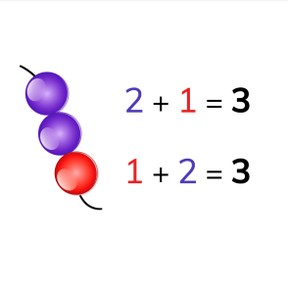I can add numbers up to 10 when the addends swap places.8,000 schools use Gynzy92,000 teachers use Gynzy1,600,000 students use Gynzy

## General

Students learn that when you add numbers to 10, that the addends can switch places without changing the sum.

## Standards

CCSS.Math.Content.K.OA.A.1/2

## Introduction

Start by decomposing numbers by using beads. Count the how many white beads there are, how many red beads, and how many there are total. Do the same with numbers using arrows. Students can also use blocks. You can ask students to decompose a few numbers that you say with the blocks that they have. An example: Decompose 5 into 4 and..... Next, ask two students to come to the front of the class and give them some erasers. For the first example, give 3 erasers to one student, and 2 erasers to the second. Ask them to calculate the total. 3+2 = 5. Now ask the students to exchange erasers. Ask students to calculate the total. Let them discover that the answer is still 5. The problem now is 2 + 3 = 5. You can repeat this a number of times (with other objects up to 10).

## Development

- The number 6 decomposes into 2 and ?
- 2+4=6. How much is 4+2?
- What happens with the sum of an addition problem when you swap the addends?

## Guided practice

Students first do addition problems with visual support, then with number bonds, and finally without visual support. They must fill in the missing number from the problem.

## Closing

Discuss with students, that it is important to be able to do this, so you can calculate quicker and easier. To close, do the addition problems shown on the interactive whiteboard as a class. Repeat with the last exercises.

## Teaching tips

Students who have difficulty with this, can make use of manipulatives like MAB blocks (or a rekenrek). Have them decompose the number 8, for example, into 3+5. Have them set out the blocks and count how many there are together. Then swap the addends, so they work with 5+3.

## Instruction materials

Erasers, blocks/manipulatives

### The online teaching platform for interactive whiteboards and displays in schools

• Save time building lessons

• Manage the classroom more efficiently

• Increase student engagement